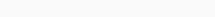# Math Puzzles Puzzle 54 Answer AndroidMath puzzle game level 54 solution with detailed hints and explanation, Scroll below to find out.

Math puzzles increase your logical thinking. The objective of the game is to find patterns hidden in the puzzle in order to arrive at the solution. Challenge yourself with different levels of math puzzles and stretch the limits of your intelligence.

### Math Puzzles Puzzle 54:

1 12 22

3 18 33

4 24 44

? 30 55Solution/Hint:

2nd Digit/First digit =6

3rd digit/fist digit=11

so

30/5=6, 55/5=11

If there is any Doubt or you are unable to understand the solution, then please let me know in comments, I will surely help you.

### One thought on “Math Puzzles Game Level 54 Answer with Solution”

•January 1, 2023 at 10:07